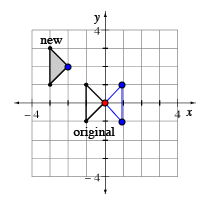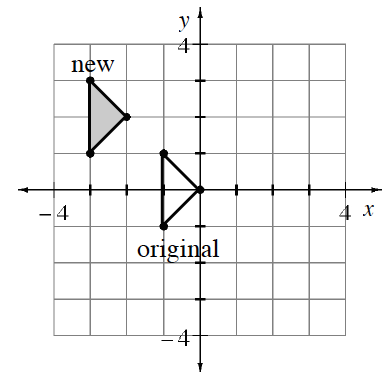### Home > CC2MN > Chapter 6 > Lesson 6.2.4 > Problem6-80

6-80.

On your paper, sketch the graph below.

1. Write directions for translating the original triangle to make the new triangle.

Describe how to get from the original points to the new points.

Move the original triangle up $2$ and to the left $2$.

1. What are the coordinates of the vertices (corners) of the new shape?

The coordinates of one of the new points are $(−2,2)$.
Can you find the coordinates of the other two vertices?

2. On your graph, reflect the original triangle over the $y$-axis. What are the coordinates of the vertices of the new triangle?

The original triangle has been reflected across the $y$-axis for you. Can you find the coordinates of this triangle's vertices?